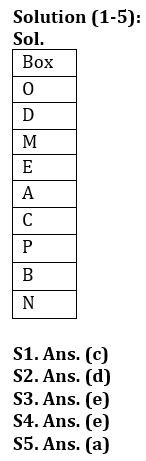# LIC ADO Prelims Reasoning Quiz 2023 – 8 March

Subject: Puzzle, Series

Directions (1-5): Study the given information carefully and answer the questions given below-
Nine boxes are placed on top of each other in a stack. No more than three boxes are placed below box C. Three boxes are placed in box C and box D. Box O is placed just above Box D. Box P is placed above box B and below box E. Box N is placed at the bottom. Box A is placed above Box C and below Box E.
Box N and Box M contain more than four boxes. Neither box A nor box M is placed at the top of the stack. Box M and Box A contain no more than one box.

Q1. Which of the following boxes is placed just above box C?
(a) Box B
(b) Box p
(c) Box A
(d) Box n
(e) None of these

Q2. Which box is placed immediately below box P?
(a) Box n
(b) Box C
(c) Box M
(d) Box B
(e) None of these

Q3. How many boxes are placed in box D and box B?
(a) One
(b) Four
(c) Two
(d) Three
(e) More than four

Q4. Four out of five of the following are based on a particular group, which one is not in that group?
(a) EC
(b) PN
(c) MA
(d) OM
(e) DP

Q5. Which box is placed at the top of the following stack?
(a) Box O
(b) Box d
(c) Box M
(d) Box p
(e) None of these

Directions (6-10): Study the following arrangement carefully and answer the questions given below:

2 3 5 7 1 2 6 4 5 7 1 9 8 5 2 4 1 4 5 8 2 6 3 7 6 9 4 1 5 6 7

Q6. Which of the following numbers will be seventh to eleventh right from left end?
(a) 2
(b) 4
(c) 5
(d) 7
(e) None of these

Q7. How many numbers less than or equal to 5 are followed by 2 in the series?
(a) One
(b) None
(c) Three
(d) Two
(e) More than three

Q8. What will be the sum of the fourth and twelfth digits of the right end and the eleventh digit of the left end?
(a) 12
(b) 11
(c) 13
(d) 10
(e) None of these

Q9. If all 2 and 4 are omitted from the series, which of the following will be fifth to the right of the twelfth digit from the left end?
(a) 7
(b) 3
(c) 8
(d) 6
(e) None of these

Q10. How many series of 7 are there that are preceded by an odd number and immediately followed by an even number?
(a) One
(b) None
(c) Three
(d) Two
(e) More than three

Directions (11-15): The following questions are based on the following five three-digit numbers:

924 574 456 187 675

Q11. If 3 is added to the second digit of each number, how many of the resulting numbers will be divisible by 3?
(a) None
(b) one
(c) Two
(d) Three
(e) None of these

Q12. If all the digits of all the numbers are arranged in descending order in the number, which of the following numbers will be the largest in the new arrangement of numbers?
(a) 924
(b) 574
(c) 456
(d) 187
(e) 675

Q13. If the third digit of the second lowest number is divided by the second digit of the second lowest number, what will be the resulting number?
(a) 2
(b) 3
(c) 0
(d) 1
(E) 4

Q14. If 3 is added to the first digit and 1 is added to the last digit of each number, which of the following numbers will be the second highest?
(a) 924
(b) 574
(c) 456
(d) 187
(e) 675

Q15. If the first and third digits of each number are interchanged, which number will be the highest?
(a) 924
(b) 574
(c) 456
(d) 187
(e) 675

SolutionSolutions (6-10):
S6. A. (b)
Sol.

Seventh factor to right of eleventh from left end (11+7) = 18th from left end is ‘4’.

S7. A. (d)
Sol. There are only two 2’s in the series followed by a number, which is less than or equal to 5, i.e. ‘2 3’ and ‘2 4’.

S8. A. (d)
Sol.

Sum of fourth and twelfth terms from right = (1+8) = 9.
Leftmost eleventh digit = 1
Hence, sum = (9+1) = 10

S9. A. (a)
Sol.

2 and 4 is after removal
3 5 7 1 6 5 7 1 9 8 5 1 5 8 6 3 7 6 9 1 5 6 7
Then, the fifth element to the right of the twelfth from the left end is ‘7’.

Sol.

There are only three 7’s that are immediately followed by an odd number, namely ‘5 7 1’, ‘5 7 1’ and ‘3 7 6’.

Solutions (11-15):